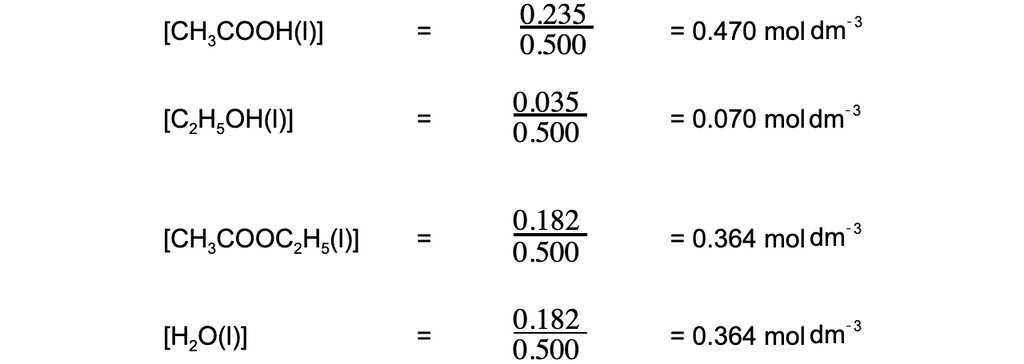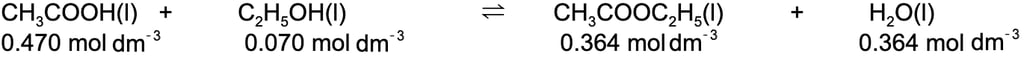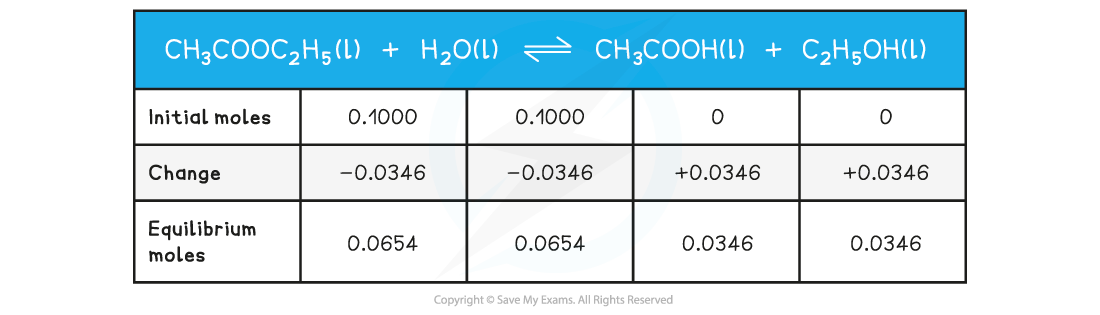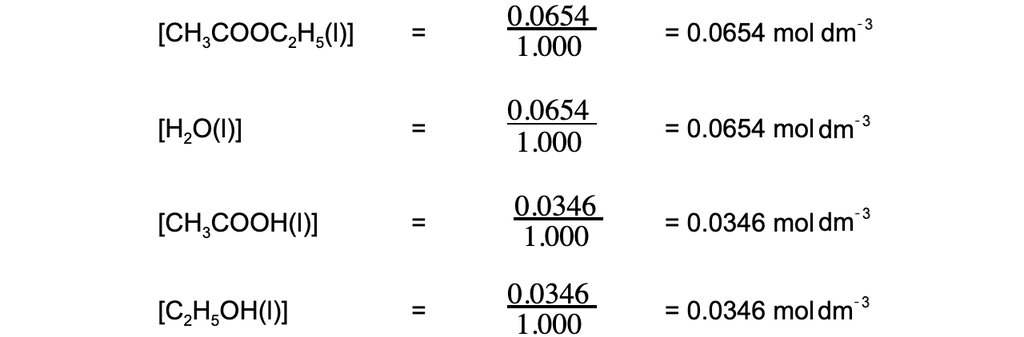# AQA A Level Chemistry复习笔记1.8.4 Calculations Involving the Equilibrium Constant

### Kc Calculations

#### Calculations involving Kc

• In the equilibrium expression each figure within a square bracket represents the concentration in mol dm-3
• The units of Kc therefore depend on the form of the equilibrium expression
• Some questions give the number of moles of each of the reactants and products at equilibrium together with the volume of the reaction mixture
• The concentrations of the reactants and products can then be calculated from the number of moles and total volumeEquation to calculate concentration from number of moles and volume

#### Worked Example

Ethanoic acid and ethanol react according to the following equation:

CH3COOH (I) + C2H5OH (I) ⇌ CH3COOC2H5 (I) + H2O (I)

At equilibrium, 500 cm3 of the reaction mixture contained 0.235 mol of ethanoic acid and 0.035 mol of ethanol together with 0.182 mol of ethyl ethanoate and 0.182 mol of water.

Calculate the value of Kc for this reaction.

Step 1: Calculate the concentrations of the reactants and productsStep 2: Write out the balanced chemical equation with the concentrations of beneath each substanceStep 3: Write the equilibrium constant for this reaction in terms of concentrationStep 4: Substitute the equilibrium concentrations into the expressionStep 5: Deduce the correct units for KcAll units cancel out

Therefore, Kc = 4.03

Note that the smallest number of significant figures used in the question is 3, so the final answer should also be given to 3 significant figures

• Some questions give the initial and equilibrium concentrations of the reactants but not the products
• An initial, change and equilibrium table should be used to determine the equilibrium concentration of the products using the molar ratio of reactants and products in the stoichiometric equation

#### Worked Example

Calculating Kc of ethyl ethanoate

Ethyl ethanoate is hydrolysed by water:

CH3COOC2H5(I) + H2O(I) ⇌ CH3COOH(I) + C2H5OH(I)

0.1000 mol of ethyl ethanoate are added to 0.1000 mol of water. A little acid catalyst is added and the mixture made up to 1dm3. At equilibrium 0.0654 mol of water are present. Use this data to calculate a value of Kc for this reaction.

Step 1: Write out the balanced chemical equation with the concentrations of beneath each substance using an initial, change and equilibrium tableStep 2: Calculate the concentrations of the reactants and productsStep 3: Write the equilibrium constant for this reaction in terms of concentrationStep 4: Substitute the equilibrium concentrations into the expression= 0.28

Step 5: Deduce the correct units for KcAll units cancel out

Therefore, Kc= 0.28